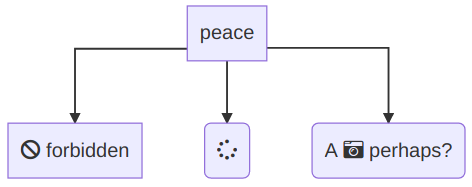Yash Singh# MermaidJS Default Curves

In this article I will share a list of curves that you can interpolate to the MermaidJS flowchart link style and a screenshot and description for each one.

## What is MermaidJS?

MermaidJS is a markdownish language allowing you to write diagrams. It is built in JavaScript and the code is parsed using Jison.

## What is interpolation in flowcharts?

Interpolation is a feature added in 6.0.0 that allows you to specify how curves should be made inside flowcharts. The syntax is like:

``linkStyle default|[...num] interpolate curveStyle [styles]``

The num represents all numbers or indexes of arrows to style. The interpolate represents that their curve style has to be changed. After that, comes the curve style and following that comes CSS styles.

The following would add the d3 curveMonotoneX on all arrows that are curving.

``````graph TD
B["peace"]
B-->C[fa:fa-ban forbidden]
B-->D(fa:fa-spinner);
B-->E(A fa:fa-camera-retro perhaps?);

It would look something like: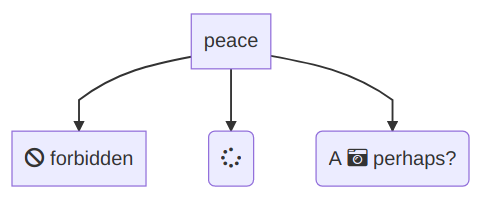Writing `monotoneX` and `MonotoneX` would both be valid because internally, MermaidJS updates the curve interpolation type to its capatilized version and precedes it with `"curve"` and finds the d3 curve type for that.

## What are the possible interpolations?

• Basis

This curve is the default curve used by MermaidJS. It has some sort of line then it curves near the end. Here is an example: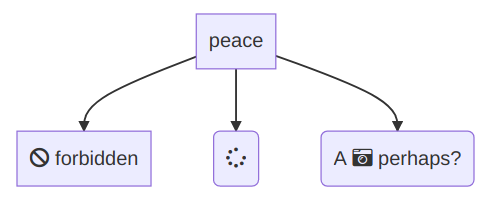• Linear

This is the fallback curve used by MermaidJS that runs when the given interpolation is invalid. It doesn't involve any curves, it just combines one or more lines to reach the end node. Here is an example: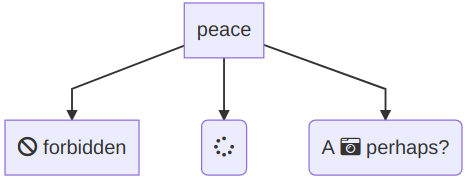• Basis Closed

This is a pretty rarely used curve. It just draws an ellipse or loop with a triangle arrowhead at some point on it. Here is an example: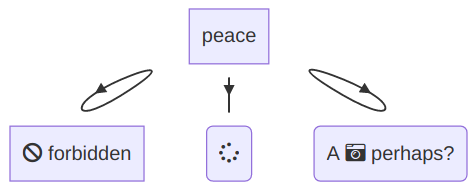• Linear Closed

The linear closed curve draws a triangle pointing towards the node. Here is how it looks: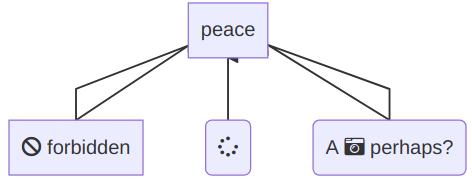• Monotone X

This curve is just a curve then another straighter curve. It looks like this:• Monotone Y

The Monotone Y curve is a line, a curve at the turning point, then a line. It looks something like this: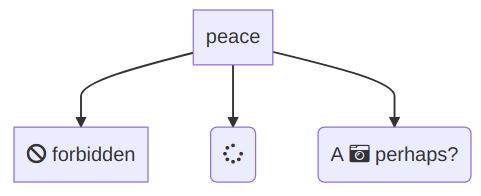• Natural

The natural curve is a pure curve and it sort of slides out and then comes back in. Here is an example of how it looks: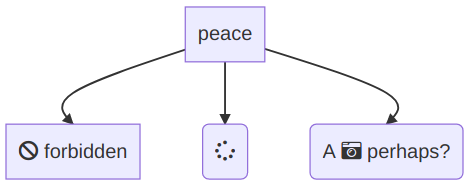• Step

The step curve is formed of linear lines that make their way to the end node. The lines are either vertical or horizontal and aren't diagonal. It sort of forms a stairsteps. Here is how it looks: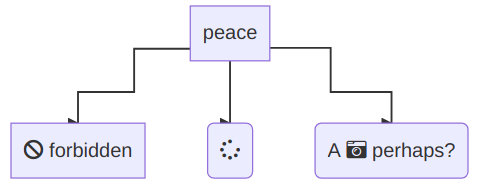• Step Before

The step before curve starts with a line going straight down (straight out in horizontal charts) instead of outwards.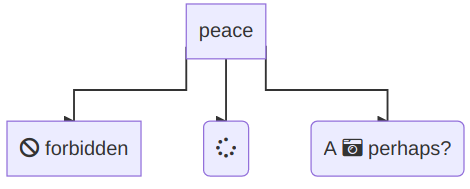• Step After

The step after curve goes out first and tries to do a minimal amount of steps. Also, the arrowhead is facing the right direction in it.